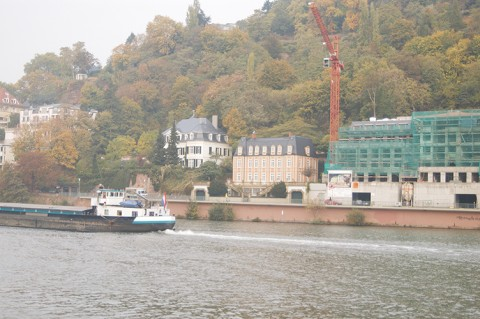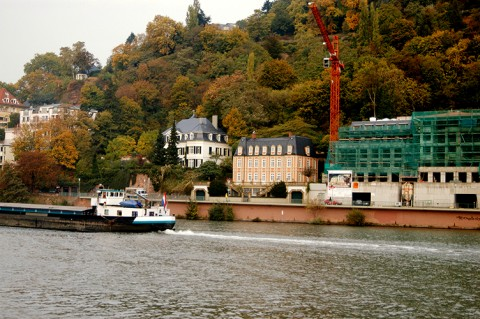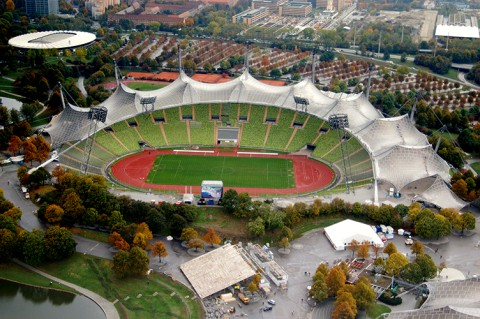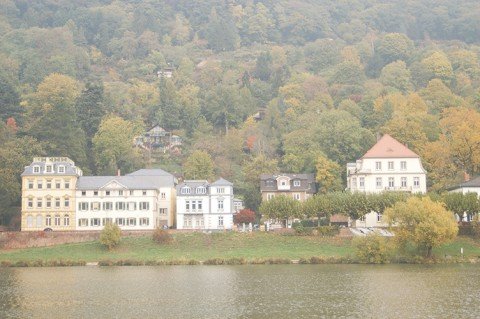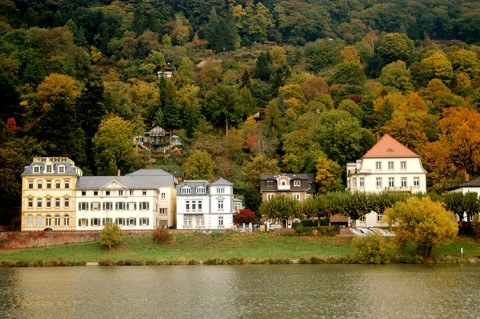Poisson Matting

 Jian Sun Jiaya Jia Chi-Keung Tang Heung-Yeung Shum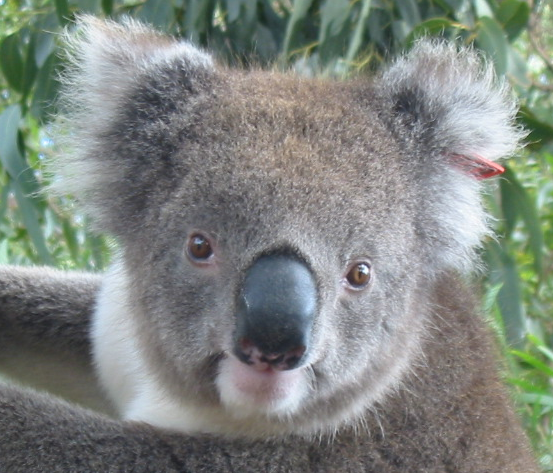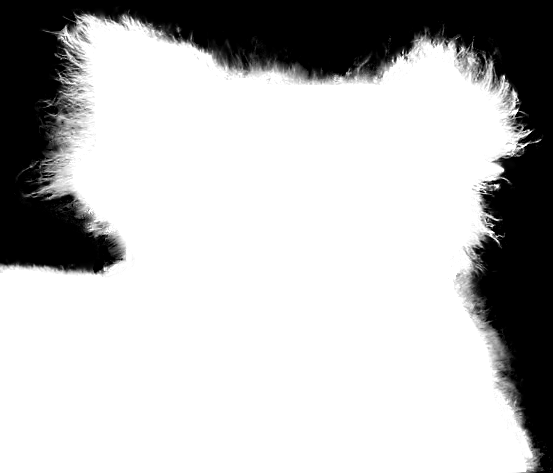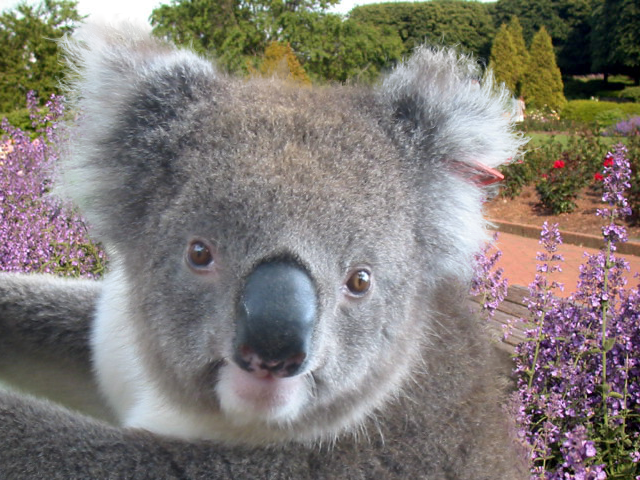Abstract ― In this paper, we formulate the problem of natural image matting as one of solving Poisson equations with the matte gradient field. Our approach, which we call Poisson matting, has the following advantages. First, the matte is directly reconstructed from a continuous matte gradient field by solving Poisson equations using boundary information from a user-supplied trimap. Second, by interactively manipulating the matte gradient field using a number of filtering tools, the user can further improve Poisson matting results locally until he or she is satisfied. The modified local result is seamlessly integrated into the final result. Experiments on many complex natural images demonstrate that Poisson matting can generate good matting results that are not possible using existing matting techniques.

Publications:

BibTex:

@article{poisson_matting,
author = {Jian Sun and Jiaya Jia and Chi-Keung Tang and Heung-Yeung Shum},

title = {Poisson matting},
journal = {ACM Transactions on Graphics},
year = {2004},
volume = {23},
number = {3},
}

Basic analysis: gradient manipulation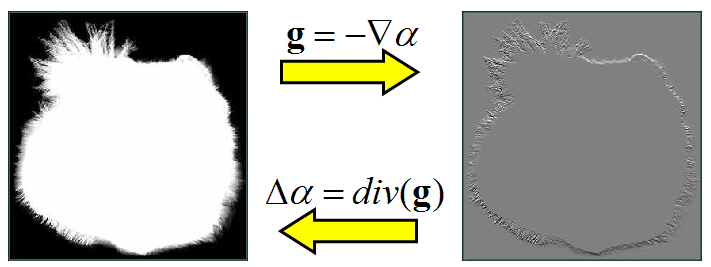Basic formula: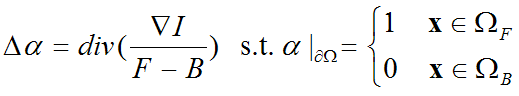Global Poisson results:

Local Poisson results:

De-fogging:

 Input images De-fogged images